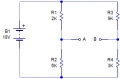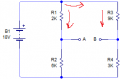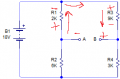# KVL orientation question

#### Transatlantic

Joined Feb 6, 2014
44I was following an example that was using KVL to calculate the Voltage across A/B.

The way they did it was to assume the current was flowing like this :Vr1 = 4.5v (using the R1/R2 divider)
Vr3 = 13.5v (using the R3/R4 divider)

And then calculate the top right loop using KVL, going clockwise :

Vr3 + Vab - Vr1 = 0
Vab = -Vr3 + Vr1
Vab = -13.5 + 4.5
Vab = -9v

I've been reading though, that if you don't know the current direction, you can just assume a direction, and that as long as you stay consistant, the value will still come out correct. You'll just have to remember the direction is actually flipped if you get a negative current.

So I thought I'd try a different current direction.But I get a different result using KVL :

Vr3 + Vab + Vr1 = 0
Vab = Vr3 + Vr1
Vab = 13.5 + 4.5
Vab = 18v

Have a misunderstood something here? .. I appreciate that in the above example the currenly is clearly going the other way, but I wanted to just try a different direction as I understood the direction could be arbitrary?

#### ericgibbs

Joined Jan 29, 2010
17,151
hi T,
Why is that upper arrow in your circuits at that location.?
Move the arrow to the left of node 'X', is the assumed direction of current flow consistent for all the circuit.????

E

#### Picbuster

Joined Dec 2, 2013
1,041
Calculating this type of circuits;
always calculate from one pole of battery normally the minus pole.
Volt A from -batt = R2 * 18/(r2+r1)
Volt B from -batt = R4 * 18/(r4+r3)
The voltage between A and B= |(Volt A-volt B) I took the absolute value reason volt A is, in this case, higher then Volt B but don't know where A and B are connected to so I defined the potential difference.

Picbuster

#### Rodney Phillips

Joined Jun 12, 2015
11
Vab would be equal to the sum of Vr2 and Vr4, not Vr1 and Vr3.

#### Dodgydave

Joined Jun 22, 2012
10,685
6/8 x 18v = A

3/12 x 18v = B,

subtract the difference, 9V,,, simple ohms law.

#### Transatlantic

Joined Feb 6, 2014
44
6/8 x 18v = A

3/12 x 18v = B,

subtract the difference, 9V,,, simple ohms law.
I think you missed the point. I was trying to calculate it using KVL, which lead to the current direction question.

#### Dodgydave

Joined Jun 22, 2012
10,685
I think you missed the point, A is more positive than B,.

#### dl324

Joined Mar 30, 2015
15,511
I was trying to calculate it using KVL, which lead to the current direction question.
Where in KVL does it state you can assume arbitrary current directions?

#### Transatlantic

Joined Feb 6, 2014
44
Where in KVL does it state you can assume arbitrary current directions?

"The directions for the currents were chosen arbitrarily. Keep in mind that there is no "wrong" direction when choosing the direction of the arrow, as long as we are consistent with each current's direction."

It is my understanding that when using KVL to sum the voltages around a loop, you need to know whether to add or subtract the voltage at a certain segment in the loop.

"It doesn't matter if you go clockwise or counter-clockwise. If you go from a "+" sign to a "-" sign when going around the loop, subtract the voltage across that element. Otherwise, add it. Once you've completed the loop, set the summation equal to zero".

I thought you used the current direction to figure out if it's a + or - you are going through.

#### dl324

Joined Mar 30, 2015
15,511

#### Transatlantic

Joined Feb 6, 2014
44
That was taken from the section for KCL.
But how have you decided on the orientation of the '+' '-' at each point in your example? Thats the part I don't understand.

I thought you work them out from the current direction?

#### dl324

Joined Mar 30, 2015
15,511
But how have you decided on the orientation of the '+' '-' at each point in your example? Thats the part I don't understand.
I used the voltage divider equation to calculate the voltage across R2 and R4. That established the voltages at A and B.

#### Transatlantic

Joined Feb 6, 2014
44But take an example like that. Why have they chosen the + to be on the left of R2? Why isn't it on the right and the - on the left? How is it determined?

#### dl324

Joined Mar 30, 2015
15,511
They made arbitrary current direction assignments and that resulted in the polarity of the drop across R2 being what it is.

#### Jony130

Joined Feb 17, 2009
5,439
The current directions determines the voltage polarity across R2 resistor (across all resistors).

#### Transatlantic

Joined Feb 6, 2014
44
Which is what I thought I did? .. I made an arbitrary current direction.Vr3 + Vab + Vr1 = 0
Vab = Vr3 + Vr1
Vab = 13.5 + 4.5
Vab = 18v

..and get the wrong answer. This is my confusion.

#### Jony130

Joined Feb 17, 2009
5,439
But how did you solve for VR1 and VR2 ??

#### Jony130

Joined Feb 17, 2009
5,439
Also did your solution for VR1 is consistent with your assumed current direction ? The answer is NO.
Because if the current is flow from point A toward upper R1 terminal VR1 is negative (-4.5V) therefore
Vab = -Vr3 - Vr1 = 13.5V - (-4.5V) = -9V

Last edited:

#### dl324

Joined Mar 30, 2015
15,511
Which is what I thought I did? .. I made an arbitrary current direction.Vr3 + Vab + Vr1 = 0
Vab = Vr3 + Vr1
Vab = 13.5 + 4.5
Vab = 18v

..and get the wrong answer. This is my confusion.
If you determine the currents at the top node, you'll find that that gives a negative current for the direction you assumed through R1 and that will reverse the polarity of the voltage drop.

And you still need to show how you determined the voltage drops.

To do it with KVL and KCL, you need to setup equations for KCL and solve the simultaneous equations.